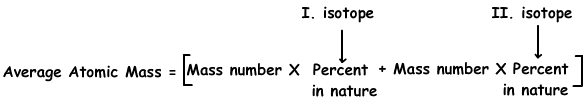EN | ES | DE | NL | RU

## Atomic Structure Exam1 and Problem Solutions

Atomic Structure Exam1 and  Problem Solutions

1. Which one of the following statements are always true for neutral atoms;

I. Atomic number is equal to number of electrons

II. Mass number is equal to sum of electrons number and neutron number

III. Atomic number is equal to neutron number

Solution:

I. In neutral atoms, number of protons is equal to number of electrons. Since atomic number is equal to number of protons we can say that atomic number is also equal to number of electrons in neutral atoms. I is true.

II. Mass number is equal to sum of number of protons and number of neutrons. Since number of protons is equal to number of electrons in neutral atoms, we can say that mass number is also equal to sum of number of electrons and neutrons. II is true.

III. In neutral atoms, number of neutrons is not always equal to number of protons. Thus, III can be true or false.

2. If  number of electrons of 37X- and 20Y+2 are equal; find number of neutrons of 37X- .

Solution:

Number of electrons of 20Y+2 ;

20-2=18

So, X- also has 18 electrons. Number of protons of 37X- is;

18-1=17

Number of neutrons of 37X- is;

37-17=20

3. Which ones of the following statements are true for 2964Cu atom;

I. Nuclear charge of Cu is 29

II. Mass number of Cu is 64

III. Number of neutrons of Cu is larger than number of protons

Solution:

Nuclear charge of atoms is equal to number of protons, so I is true.

Number written at left corner of atom shows mass number. II is true

We can find number of neutrons;

Mass number= number of protons + number of neutrons

64 = 29 + number of neutrons

number of neutrons = 35

III is also true number of neutrons is larger than number of protons.

4. Which ones of the following statements are true for 1224X and 1124Y atoms;

I. Relation between nuclear charges : X > Y

II. Relation between number of neutrons: Y = X + 1

III. Number of nucleons are equal.

Solution:

1224X has;

Mass Number=24 =number of nucleons

Atomic Number=12=number of protons =nuclear charge

Mass number = p + n

24=12 + n

n=12 number of neutrons of X

1124Y has;

Mass Number=24 =number of nucleons

Atomic Number=11=number of protons =nuclear charge

Mass number = p + n

24=11 + n

n=13 number of neutrons of Y

Thus, I, II and III are all true.

5. If S-2 ion has 18 electrons, find atomic number of S.

Solution:

S-2 ion has 18 electrons, this means that, S takes 2 electrons from outside and has 18 electrons. Thus, in neutral S atom there are;

18-2=16 electrons. For neutral atoms;

number of protons=number of electrons=atomic number

So; atomic number of S is 16.

6. Chlorine has two isotopes; 1735Cl in 75 % and 1737Cl in 25%. Find average atomic mass of Cl atom.

Solution:

We use following formula to find average atomic mass of isotopes;Average atomic mass=35.75/100 + 37.25/100

Average atomic mass=35,5 g

The Original Author: